# How to Use axis=0 and axis=1 in Pandas?

• Difficulty Level : Easy
• Last Updated : 19 Dec, 2021

In this article, we will discuss how to use axis=0 and axis=1 in pandas using Python.

Sometimes we need to do operations only on rows, and sometimes only on columns, in such situations, we specify the axis parameter. In this article, let’s see a few examples to know when and how to use the axis parameter. In pandas axis = 0 refers to horizontal axis or rows and axis = 1 refers to vertical axis or columns.

## AXIS =0

When the axis is set to zero while performing a specific action, the action is performed on rows that satisfy the condition.

Dataset Used: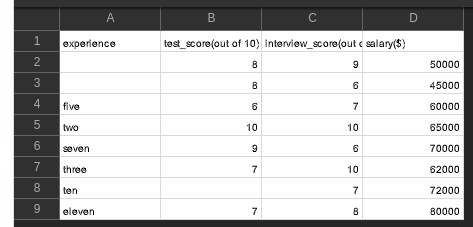Example: Using axis=0

## Python3

 `# importing packages``import` `pandas as pd`` ` `# importing our dataset``df ``=` `pd.read_csv(``'hiring.csv'``)`` ` `# dropping the column named 'experience'``df ``=` `df.drop([``0``, ``3``], axis``=``0``)`` ` `# 'viewing the dataframe``df.head()`

Output: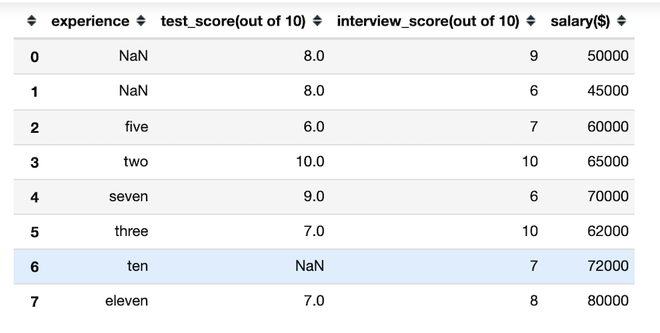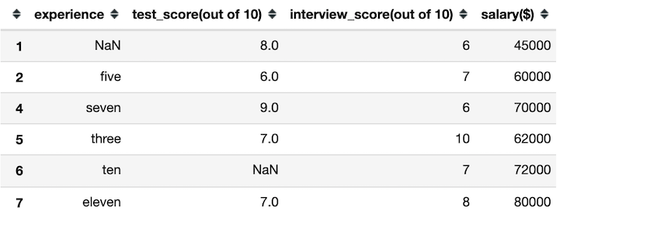Example: Using axis=0

## Python3

 `# importing packages``import` `pandas as pd`` ` `# creating a dataset``df ``=` `pd.DataFrame([[``1``, ``2``, ``3``], [``4``, ``5``, ``6``],``                   ``[``7``, ``8``, ``9``], [``10``, ``11``, ``12``]],``                  ``columns``=``[``'a'``, ``'b'``, ``'c'``])`` ` `# viewing the dataFrame``print``(df)`` ` `# finding mean by rows``df.mean(axis``=``'rows'``)`

Output:

[Image_Link]https://media.geeksforgeeks.org/wp-content/uploads/2021112

## AXIS=1

When the axis is set to one while performing a specific action, the action is performed on column(s) that satisfy the condition.

Example: Using axis=1

## Python3

 `# importing packages``import` `pandas as pd`` ` `# importing our dataset``df ``=` `pd.read_csv(``'hiring.csv'``)`` ` `# dropping the column named 'experience'``df ``=` `df.drop([``'experience'``], axis``=``1``)`` ` `# 'viewing the dataframe``df.head()`

Output: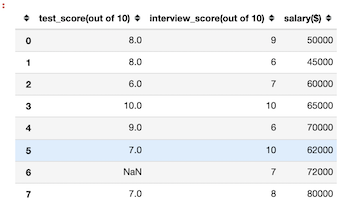Example: Using axis=1

## Python3

 `# importing packages``import` `pandas as pd`` ` `# creating a dataset``df ``=` `pd.DataFrame([[``1``, ``2``, ``3``], [``4``, ``5``, ``6``],``                   ``[``7``, ``8``, ``9``], [``10``, ``11``, ``12``]], ``                  ``columns``=``[``'a'``, ``'b'``, ``'c'``])`` ` `# viewing the dataFrame``print``(df)`` ` `# finding mean by columns``df.mean(axis``=``'columns'``)`

Output: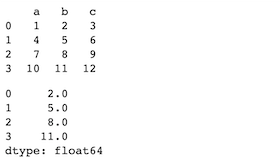My Personal Notes arrow_drop_up